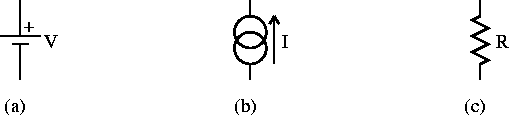Next: Electromotive Force (EMF) Up: Direct Current Circuits Previous: Resistance and Ohm's Law

# The Schematic Diagram

The schematic diagram consists of idealized circuit elements each of which represents some property of the actual circuit. Figure 1.1 shows some common circuit elements encountered in DC circuits. A two-terminal network is a circuit that has only two points of interest, say A and B.Figure 1.1:   Common circuit elements encountered in DC circuits: a) ideal voltage source, b) ideal current source and c) resistor.

Doug Gingrich
Tue Jul 13 16:55:15 EDT 1999